### Home > CAAC > Chapter 14 > Lesson 14.2.1.1 > Problem2-16

2-16.
1. Solve each of the following equations. Homework Help ✎

1.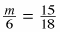2.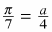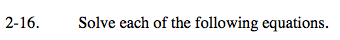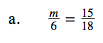Multiply both sides by 6.

$\text{6\cdot \left(\frac{m}{6}\right)=\left(\frac{15}{18}\right)\cdot 6}$

m = 5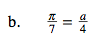See part (a).